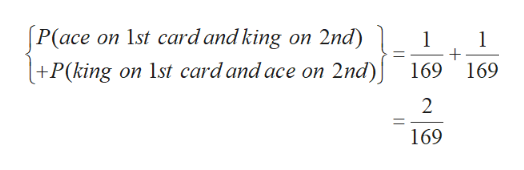# You draw two cards from a standard deck of 52 cards, but before you draw the second card, you put the first one back and reshuffle the deck.(a) Are the outcomes on the two cards independent? Why?Yes. The probability of drawing a specific second card is the same regardless of the identity of the first drawn card.No. The events cannot occur together.     Yes. The events can occur together.No. The probability of drawing a specific second card depends on the identity of the first card.(b) Find P(ace on 1st card and king on 2nd). (Enter your answer as a fraction.)(c) Find P(king on 1st card and ace on 2nd). (Enter your answer as a fraction.)(d) Find the probability of drawing an ace and a king in either order. (Enter your answer as a fraction.)

Question

You draw two cards from a standard deck of 52 cards, but before you draw the second card, you put the first one back and reshuffle the deck.

(a) Are the outcomes on the two cards independent? Why?
Yes. The probability of drawing a specific second card is the same regardless of the identity of the first drawn card.No. The events cannot occur together.     Yes. The events can occur together.No. The probability of drawing a specific second card depends on the identity of the first card.

(b) Find P(ace on 1st card and king on 2nd). (Enter your answer as a fraction.)

(c) Find P(king on 1st card and ace on 2nd). (Enter your answer as a fraction.)

(d) Find the probability of drawing an ace and a king in either order. (Enter your answer as a fraction.)
check_circleExpert Solution
Step 1

(a)

If the outcomes of the two cards are independent, then the probability of drawing a specific second card is the same regardless of the identity of the first drawn card.

Hence, the probability of drawing a specific second card is the same regardless of the identity of the first drawn card.

Thus, the correct statement is “Yes. The probability of drawing a specific second card is the same regardless of the identity of the first drawn card”.

(b)

The value of P (ace on 1st card and king on 2nd) is obtained below:

Step 2

Thus, the value of P (ace on 1st card and king on 2nd) is 1/169.

(c)

The value of P(king on 1st card and ace on 2nd) is obtained below:

Step 3

Thus, the value of P(king on 1st card and ace on 2nd) is 1/169.

(d)

The probability of drawing an...help_outlineImage TranscriptioncloseP(ace on 1st card and king on 2nd) +P(king on 1st card and ace on 2nd) 1 1 + 169 169 2 169 fullscreen

### Want to see the full answer?

See Solution

#### Want to see this answer and more?

Solutions are written by subject experts who are available 24/7. Questions are typically answered within 1 hour*

See Solution
*Response times may vary by subject and question
Tagged in

### Other